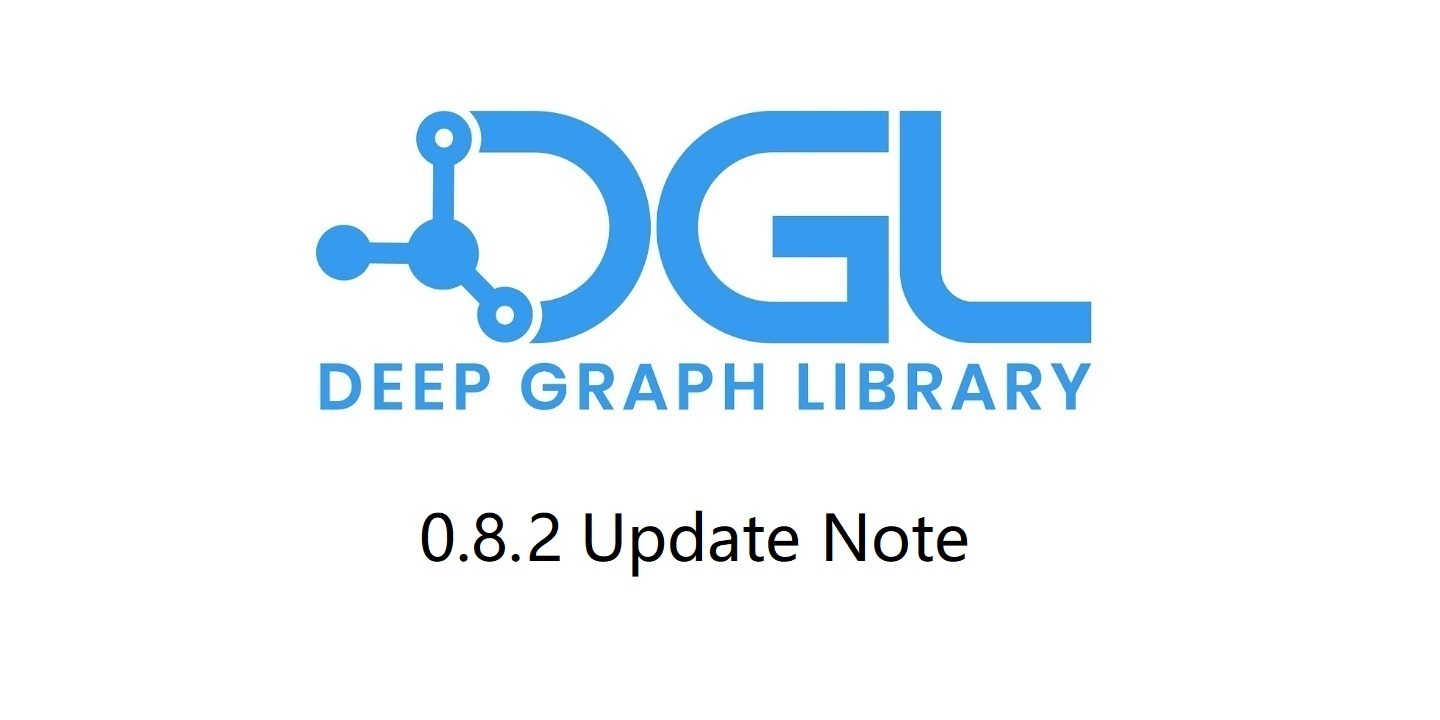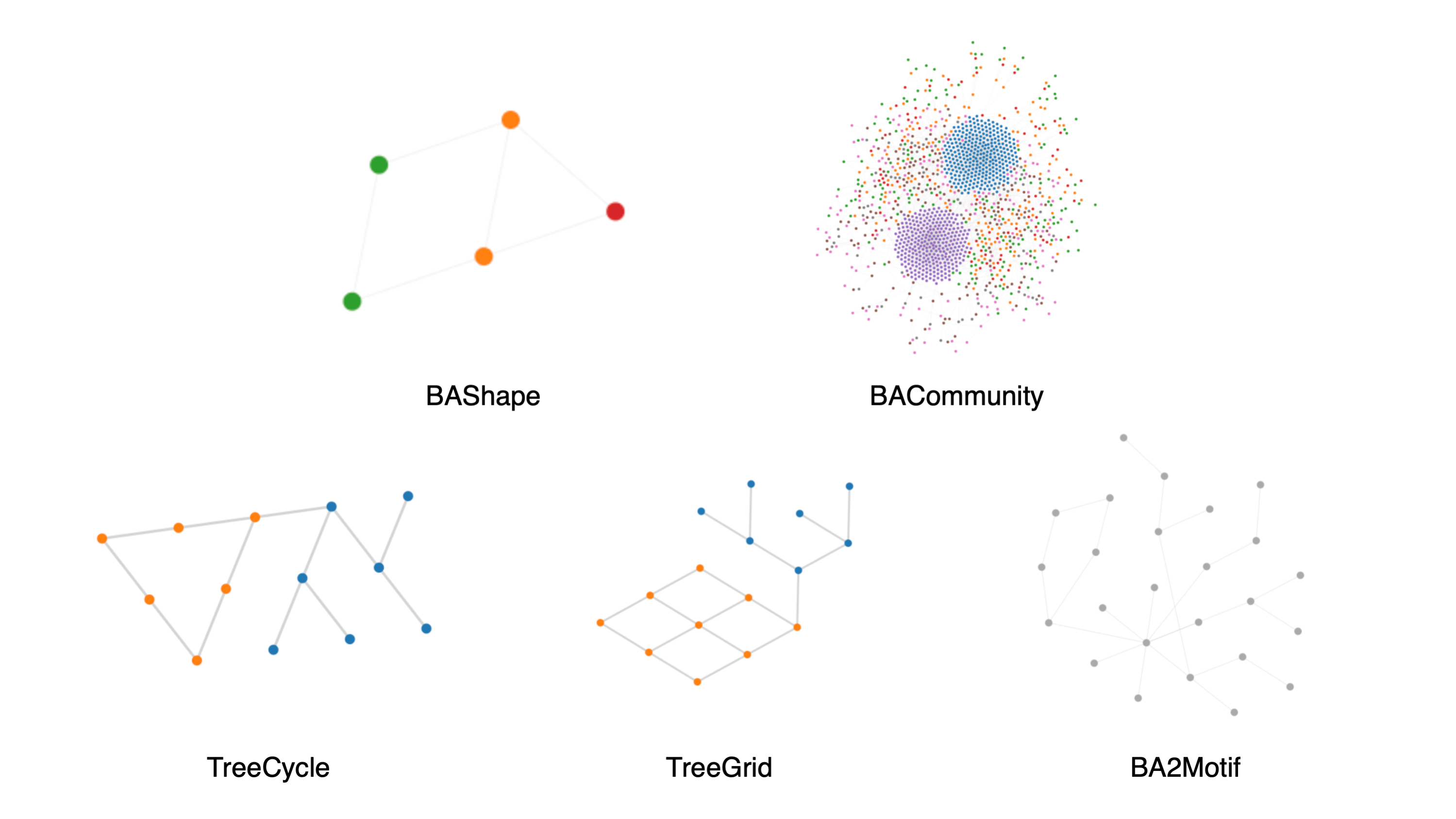## Blog Details

Home / Blog Details## Synthetic Datasets for Developing GNN Explainability Approaches

We added the following new datasets. BAShapeDataset, BACommunityDataset, TreeCycleDataset, and TreeGridDataset were first introduced in GNNExplainer: Generating Explanations for Graph Neural Networks for node classification. BA2MotifDataset was first introduced in Parameterized Explainer for Graph Neural Network for graph classification.# A dataset for node classification
from dgl.data import BAShapeDataset

dataset = BAShapeDataset()
num_classes = dataset.num_classes
graph = dataset
feat = graph.ndata['feat']
label = graph.ndata['label']

# A dataset for graph classification
from dgl.data import BA2MotifDataset

dataset = BA2MotifDataset()
num_classes = dataset.num_classes
graph, label = dataset           # Get the first graph data
feat = graph.ndata['feat']


Developer Recommendation: These synthetic graphs integrate specially designed substructures, motifs, into traditional random graph models, and assign labels based on their existence. Therefore, those substructures act as ground truth explanations for the node/graph labels, making them commonly used benchmarks for evaluating GNN explainability approaches.

## SIGN Diffusion Transform

We added a new data transform module SIGNDiffusion first introduced in SIGN: Scalable Inception Graph Neural Networks, which diffuses node features for later use. It supports four built-in diffusion matrices, including raw adjacency matrix, random walk adjacency matrix, symmetrically normalized adjacency matrix, and personalized PageRank matrix.

import dgl
import dgl.transforms as T

dataset = dgl.data.CoraGraphDataset(
transform=T.SIGNDiffusion(k=2)) # Diffuse for 1 & 2 hops
g = dataset  # diffused node features will be generated as ndata
feat1 = g.ndata['out_feat_1']       # feature diffused for 1 hop
feat2 = g.ndata['out_feat_2']       # feature diffused for 2 hops


Developer Recommendation: The ability to learn to aggregate neighbor information is one of the key innovation of Message Passing Neural Networks, which also brings scalability challenges due to the exponentially growing receptive field with more hops of neighbors to explore. SIGN proposed a cheap yet efficient solution that decouples model depth and receptive field size by diffusing input node features using various kinds of algorithms. Because the diffusion process is not trainable, we package it as a data transform module so that users can easily plug-in SIGN before running their own model.

## Label Propagation

We added a new NN module LabelPropagation first introduced in Learning from Labeled and Unlabeled Data with Label Propagation, which propagates node labels over a graph for inferring the labels of unlabeled nodes.

import dgl

lp = dgl.nn.LabelPropagation(k=3, alpha=0.9)
dataset = dgl.data.CoraGraphDataset()
g = dataset
labels = g.ndata['label']


Developer Recommendation: The classical label propagation is a simple (non-parametric) yet effective algorithm, making it a strong baseline for many node classification datasets.

## Directional Graph Network Layer

We added a new NN module DGNConv first introduced in Directional Graph Networks, which introduces directional aggregators in message passing based on the gradient of low-frequency eigenvectors of the graph Laplacian matrix.

import dgl
import torch

g = ...  # some graph
# Precompute 1 smallest non-trivial eigenvectors
transform = dgl.transforms.LaplacianPE(k=1)
g = transform(g)
x = torch.randn(g.num_nodes(), 10)  # node features
eig = g.ndata['PE']
conv = dgl.nn.DGNConv(10, 10,
aggregators=['dir1-av', 'dir1-dx'],
scalers=['identity', 'amplification'],
delta=2.5)
h = conv(g, x, eig_vec=eig)


Developer Recommendation: Directional Graph Networks (DGN) allow defining graph convolutions according to topologically-derived directional flows. It is a state-of-the-art baseline for many graph classification tasks.

## Graph Isomorphism Network Layer with Edge Features

We added a new NN module GINEConv first introduced in Strategies for Pre-training Graph Neural Networks, which extends Graph Isomorphism Network (GIN) for handling edge features.

import dgl
import torch

g = ...  # some graph
xn = torch.randn(g.num_nodes(), 10)  # node features
xe = torch.randn(g.num_edges(), 10)  # edge features
conv = dgl.nn.GINEConv(torch.nn.Linear(10, 10))
hn = conv(g, xn, xe)


Developer Recommendation: Graph Isomorphism Network with edge features has been an important baseline for many graph classification tasks such as OGB graph property datasets.

We added a new data transform module FeatMask first introduced in Graph Contrastive Learning with Augmentations, which randomly masks columns of node/edge features.

import dgl
import dgl.transforms as T

dataset = dgl.data.CoraGraphDataset(
g = dataset
feat = g.ndata['feat'] # The node feature tensor has been randomly masked.


Developer Recommendation: Randomly masking columns of features is a simple yet useful data augmentation for graph contrastive learning.

## Row-Normalizer of Features

We added a new data transform module RowFeatNormalizer, which performs row-normalization of features.

import dgl
import dgl.transforms as T

dataset = dgl.data.CoraGraphDataset(
transform=T.RowFeatNormalizer(node_feat_names=['feat']))
g = dataset
feat = g.ndata['feat'] # The node feature tensor has been row-normalized.


Developer Recommendation: Row-normalization of raw features is a useful data pre-processing step.

For further readings, check out the release note for a complete list of new additions, improvements and bug fixes. If you have questions about DGL or GNN in general, welcome to join our Slack channel. If you have specific requests on what should be included in DGL next, you can submit them on our Github or fill in this survey.